### Quantum Objects

Particle spin just smashed our classical expectations.

In classical physics, the value of physical quantities like direction of spin, momentum, and position can vary continuously. There are no rules that allow or disallow certain values.

But the quantum property we call spin behaves differently. A nuclear oven should produce neutrons in any of an infinite number of possible orientations, but it turns out there are only ever two: spinning on an axis aligned up, or spinning on an axis aligned down.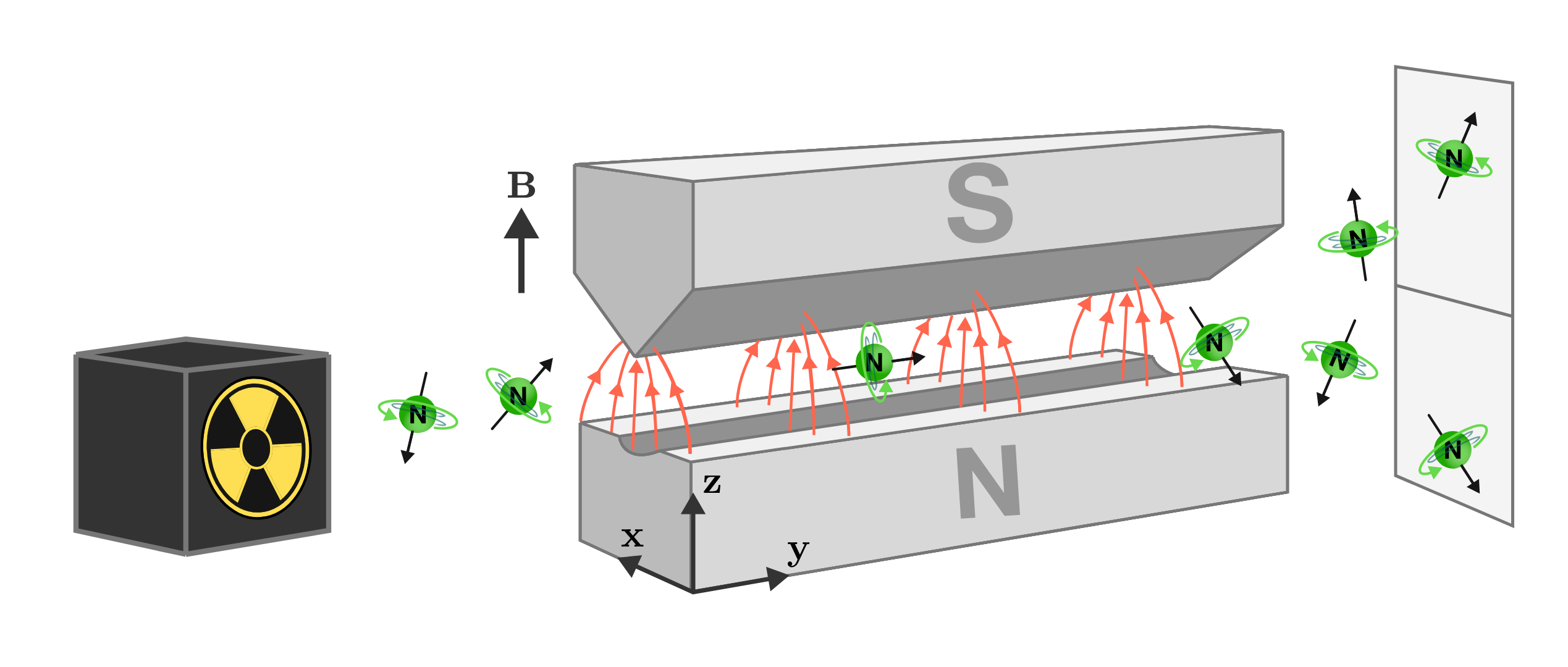Spin is our first encounter with a purely quantum property, and it was first observed experimentally using silver atoms produced in a furnace and a similar magnetic apparatus by two physicists in $1922.$ Since then it's been observed in every type of microscopic particle, including neutrons. The experimental setup we'll be using is still named after the original experimenters: Otto Stern and Walther Gerlach.

We’ve already seen that our knowledge of classical mechanics gives us limited predictive ability, so we will proceed cautiously into the dark.

# Quantum Surprises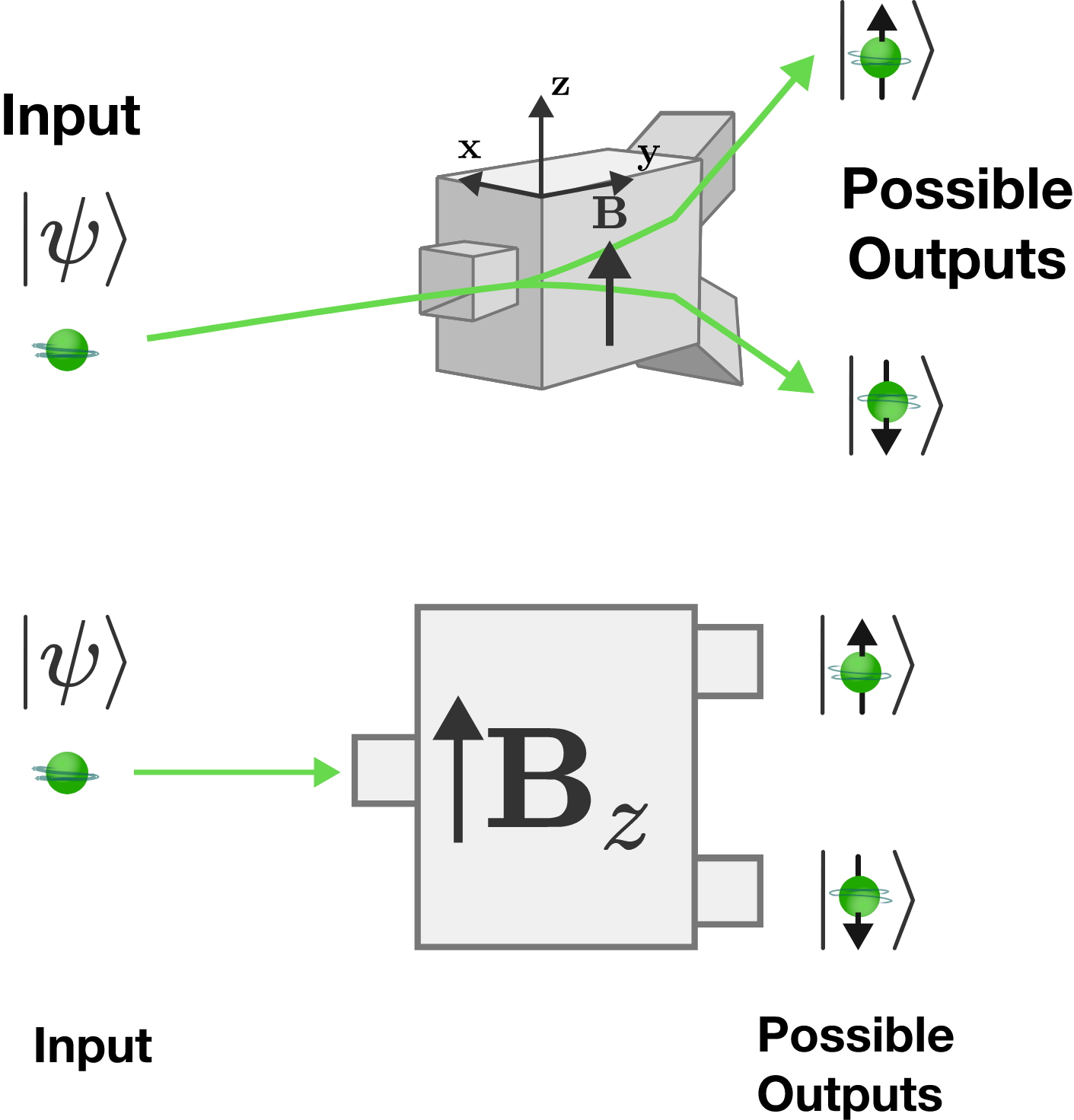The Stern-Gerlach (SG) analyzer will be a workhorse in this chapter, so let’s take a moment to clarify its operation. Stern and Gerlach's experimental device was a bit different, but we'll be able to extract all the quantum rules we need from this simple version.

The analyzer has an input for incoming particles, and only two possible output channels split by an applied magnetic field: up and down. We’ll label the analyzer $Z$ for the axis along which the magnetic field $\mathbf{B}$ is oriented.

The strange brackets around $\uparrow$ and $\downarrow$ are called “kets” and are simply a way of referring to the state of the particles in each channel. $\ket{\uparrow}$ particles have been measured to have their spin vector aligned with the positive $z$-axis, and $\ket{\downarrow}$ particles were aligned with the negative $z$-axis. The state of the particles in the input beam is unknown, so we can’t assign them a state ket like $\ket{\uparrow}$ or $\ket{\downarrow}.$ We instead refer to them using a mystery ket called $\ket{\psi}.$

In the first quiz, we chose the $z$-axis along which to measure the spin component; but there is nothing sacred about this direction in space, as we’ll soon see. We could have chosen any other axis and would have obtained the same startling binary result.

# Quantum Surprises

After picking their jaws up off the floor, and recovering the contents of their minds from around the room, the surprised Stern and Gerlach returned to the startling binary result on their analyzer to learn more. We shall do the same.

Suppose the SG analyzer has now been running for quite a while, and a very large number of neutrons had been sorted into $\ket{\uparrow}$ and $\ket{\downarrow}$ states.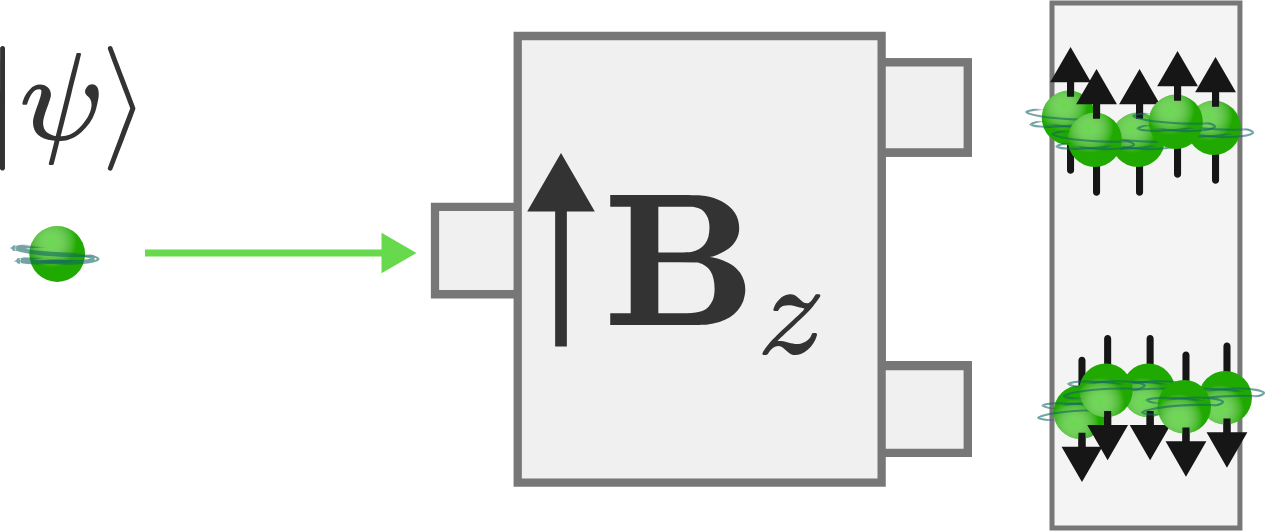Based on your understanding of classical physics, how do you expect the neutrons' states to be distributed between $\ket{\uparrow}$ and $\ket{\downarrow}?$

# Quantum Surprises

The number of neutrons in each channel of the analyzer is probabilistic: there is a $50:50$ chance for each neutron that enters the analyzer to emerge from each output.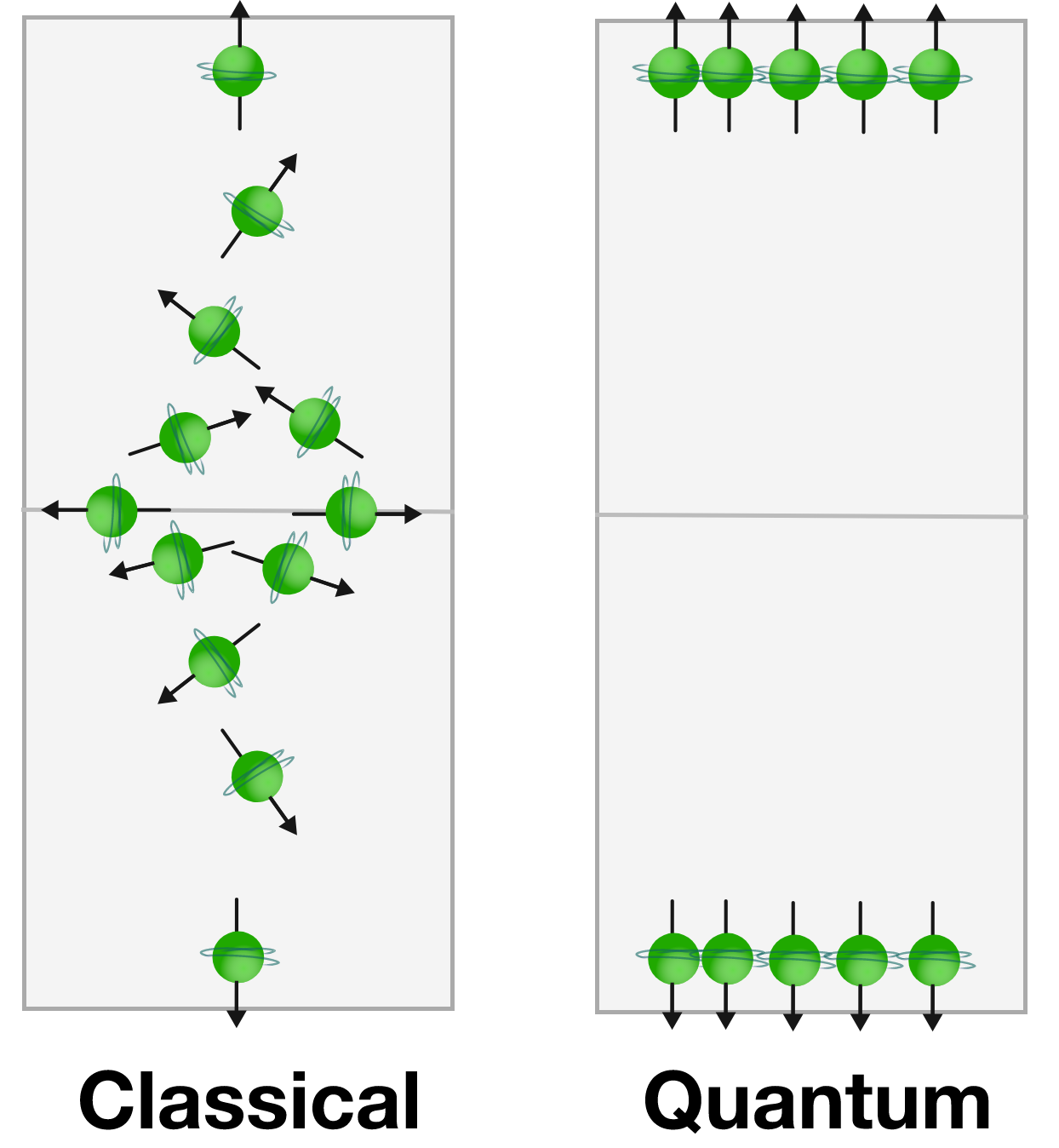Recall that based on our understanding of classical mechanics, we expected the distribution of neutron spins from our thermonuclear oven to be randomly oriented due to thermal motion in the oven. In classical mechanics, there is no preferred orientation. A lot seem to be different with quantum objects, but some remain the same. Though only two states of quantum spin are allowed, this expectation of random orientation remains.

This early correspondence between quantum and classical mechanics hints at a connection that will be seen time and again throughout this course. What quantitative conclusion can we make from comparing the classically expected and quantum distributions?

# Quantum Surprises

Probabilistic behavior is at the heart of quantum mechanics. Unsurprisingly, this behavior is one of the problems that many early theoretical and experimental physicists alike had with the theory. Many physicists argued that since we cannot predict from which output a given neutron will exit, there must simply be variables that remain unknown to us. They assumed that given complete knowledge of the system, we’d be able to deterministically predict the path of each neutron.

After much hemming and hawing over the past century, most physicists have decided that a certain amount of unpredictability is a fact of nature, though this point remains one of the biggest questions at the foundations of quantum mechanics.

# Quantum Surprises

Let’s extend the experiment a bit further.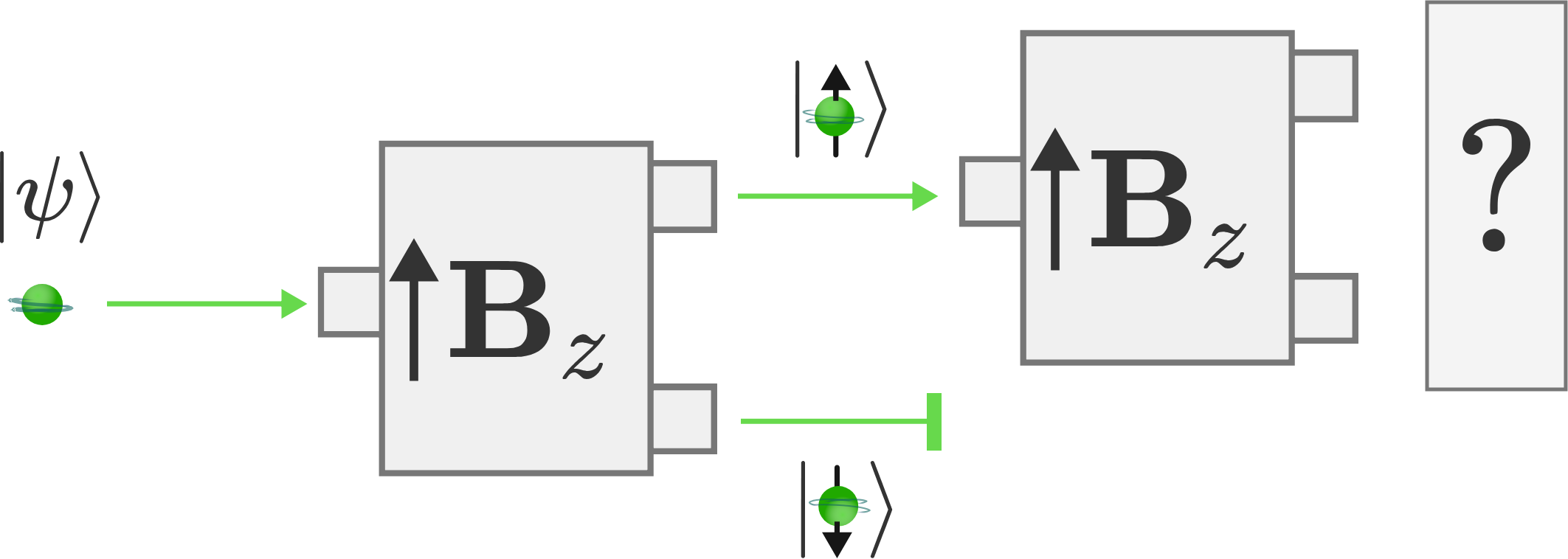Suppose we place a second SG analyzer with its input aligned with the $\ket{\uparrow}$ channel of the first analyzer. So after observing the neutrons to have a spin axis aligned with the positive $z$-axis and assigning them the state $\ket{\uparrow}$, the neutrons proceed directly into a second SG analyzer which performs an identical measurement.

What do you expect the distribution of neutrons to look like emerging from the $\ket{\uparrow}$ and $\ket{\downarrow}$ exits of the second analyzer?

# Quantum Surprises

We can all breathe a small sigh of relief: the state of the neutrons which emerge from the $\ket{\uparrow}$ channel of the first analyzer remains consistently in the $\ket{\uparrow}$ channel through the second analyzer. Though the first measurement of the unknown $\ket{\psi}$ neutrons seemed to be completely random and binary, once the neutrons have been observed in one state, they appear to remain in it.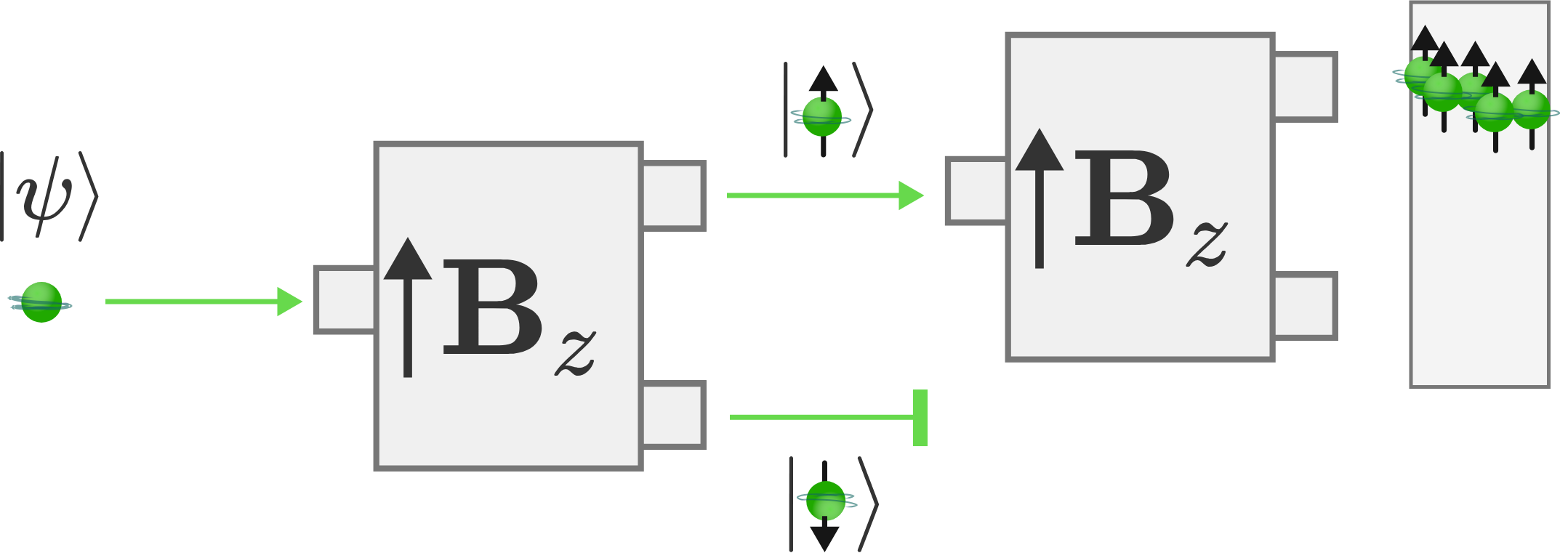Indeed, this result doesn’t just hold for two SG analyzers in series: the same result will be true for any number of repetitions. Given no other interfering magnetic fields or forces, the $\ket{\uparrow}$ state which is prepared by the first SG analyzer is robust to subsequent measurement by other analyzers.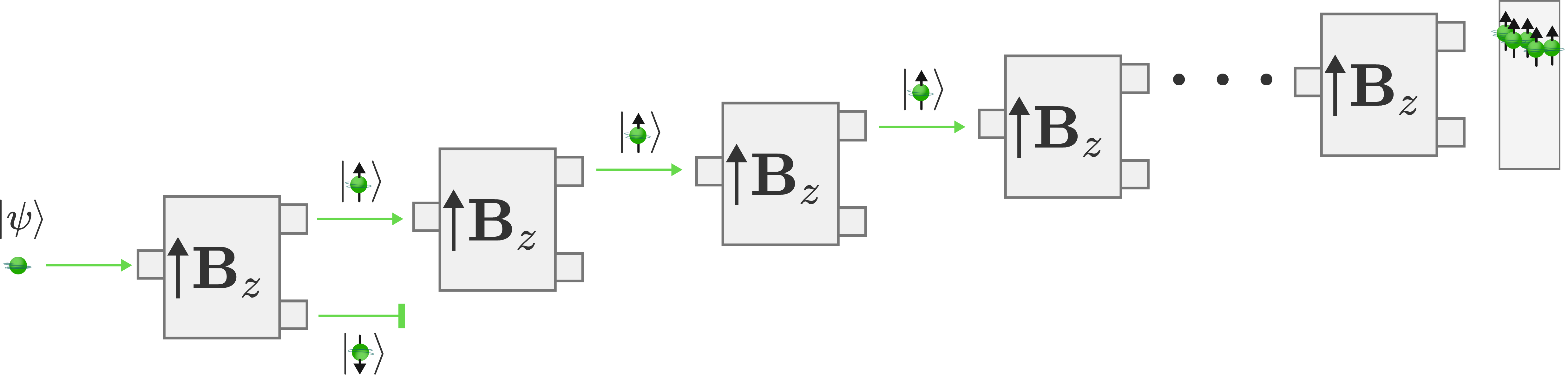# Quantum Surprises

Our physical understanding of the $\ket{\uparrow}$ neutron channel is quite simple: these neutrons have had the $z$-component of their spin axis measured, and they were found to align exactly with the magnetic field along the $z$-axis. These particles are usually referred to as having “spin up”, while the $\ket{\downarrow}$ neutrons are referred to as having “spin down”.

But spin is a quantum property. Do we actually think that these particles are spinning like tops as they proceed through the magnetic fields of the SG devices? Stern and Gerlach had the same misgivings about referring to this quantum property as spin, so they devised a very simple alteration to their experiment.

If this model of spinning neutrons is correct, what channel would you expect the neutrons to emerge from if we flipped the magnetic field in the second SG device upside-down?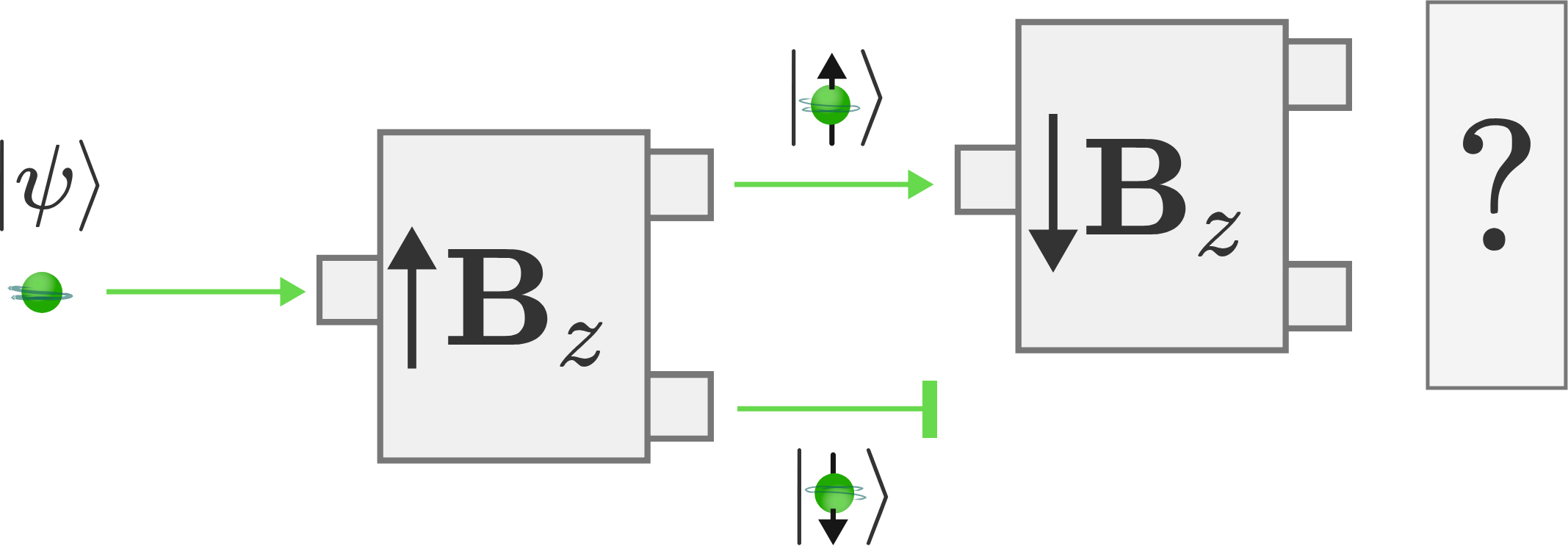# Quantum Surprises

This leaves us with a physical model that is consistent with a neutron beam acting as a stream of subatomic spinning tops, each of which creates a magnetic moment along their axis of spin just like a classical vector quantity.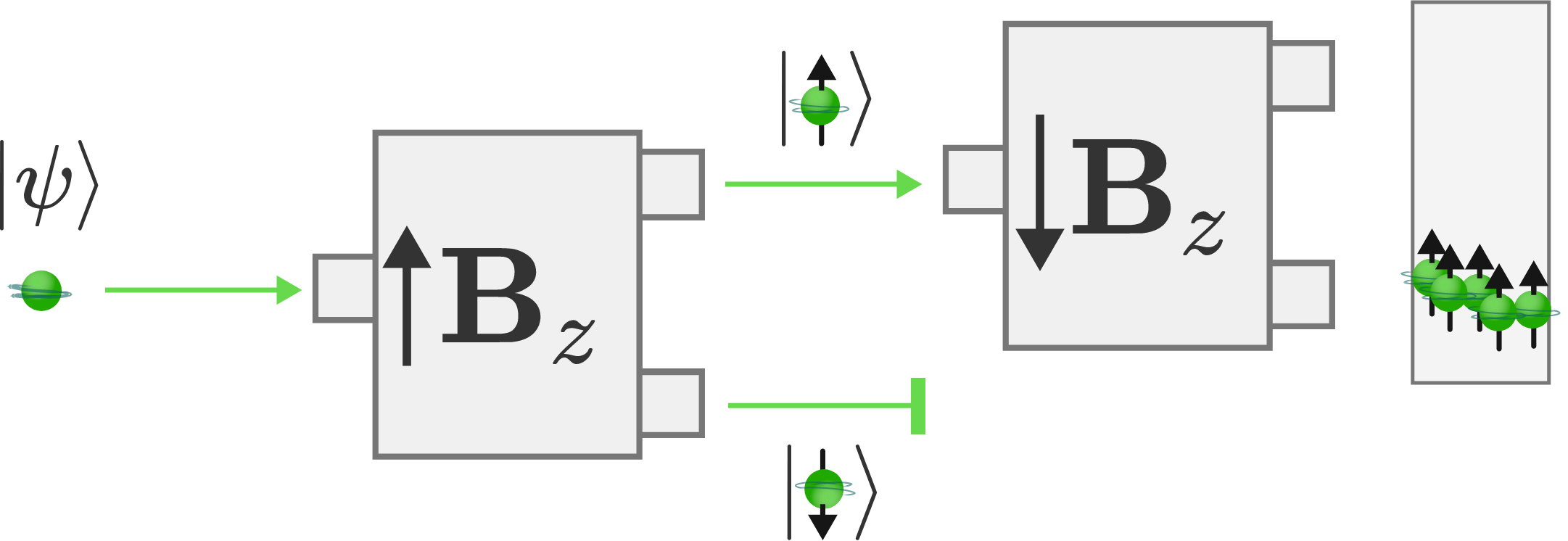This physical insight into the nature of spin gained from flipping over an experimental apparatus inspired Stern and Gerlach: what if we rotated the magnetic field by a different angle?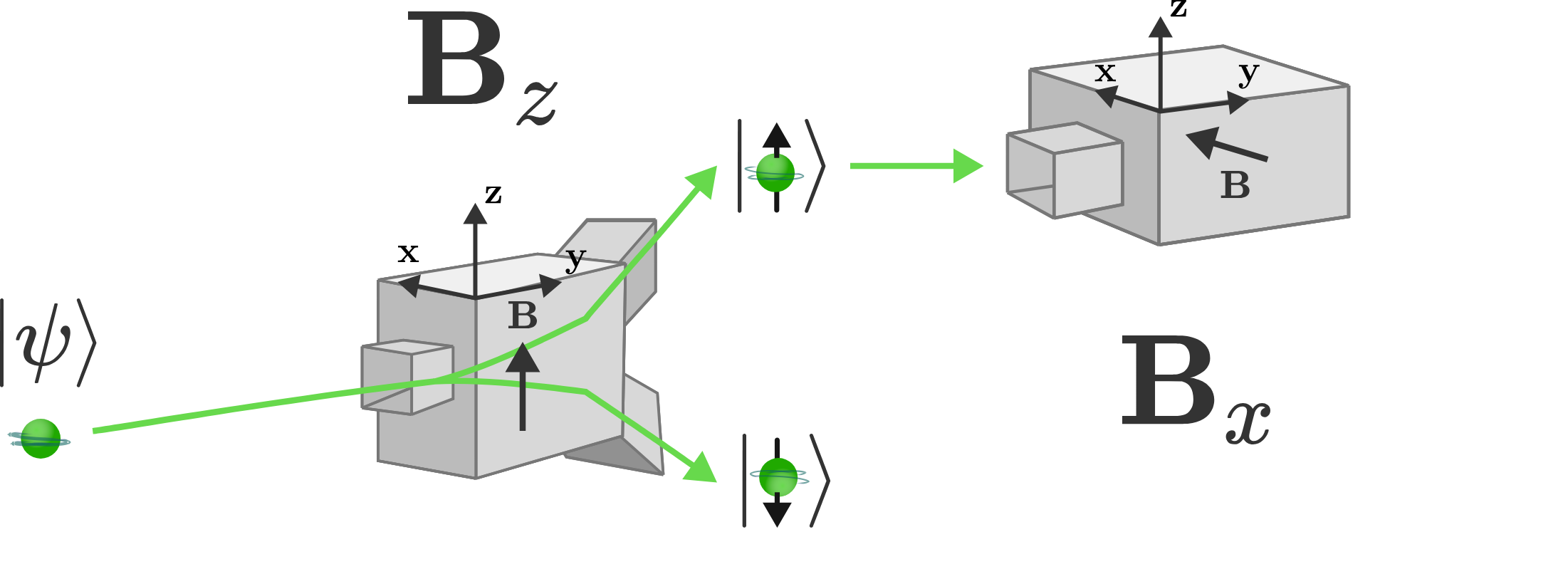# Quantum Surprises

×